# Selina Solutions Concise Maths Class 10 Chapter 2 Banking (Recurring Deposit Accounts) Exercise 2(B)

Computing the maturity value of a Recurring Deposit Account is the key focus in this exercise. For students willing to strengthen their conceptual knowledge, the Selina Solutions for Class 10 Maths is the right place to find all the solutions to problems of Class 10. Further, students can download Selina Solutions Concise Maths Class 10 Chapter 2 Banking (Recurring Deposit Accounts) Exercise 2(B) PDF, from the links provided below.

## Selina Solutions Concise Maths Class 10 Chapter 2 Banking (Recurring Deposit Accounts) Exercise 2(B) Download PDF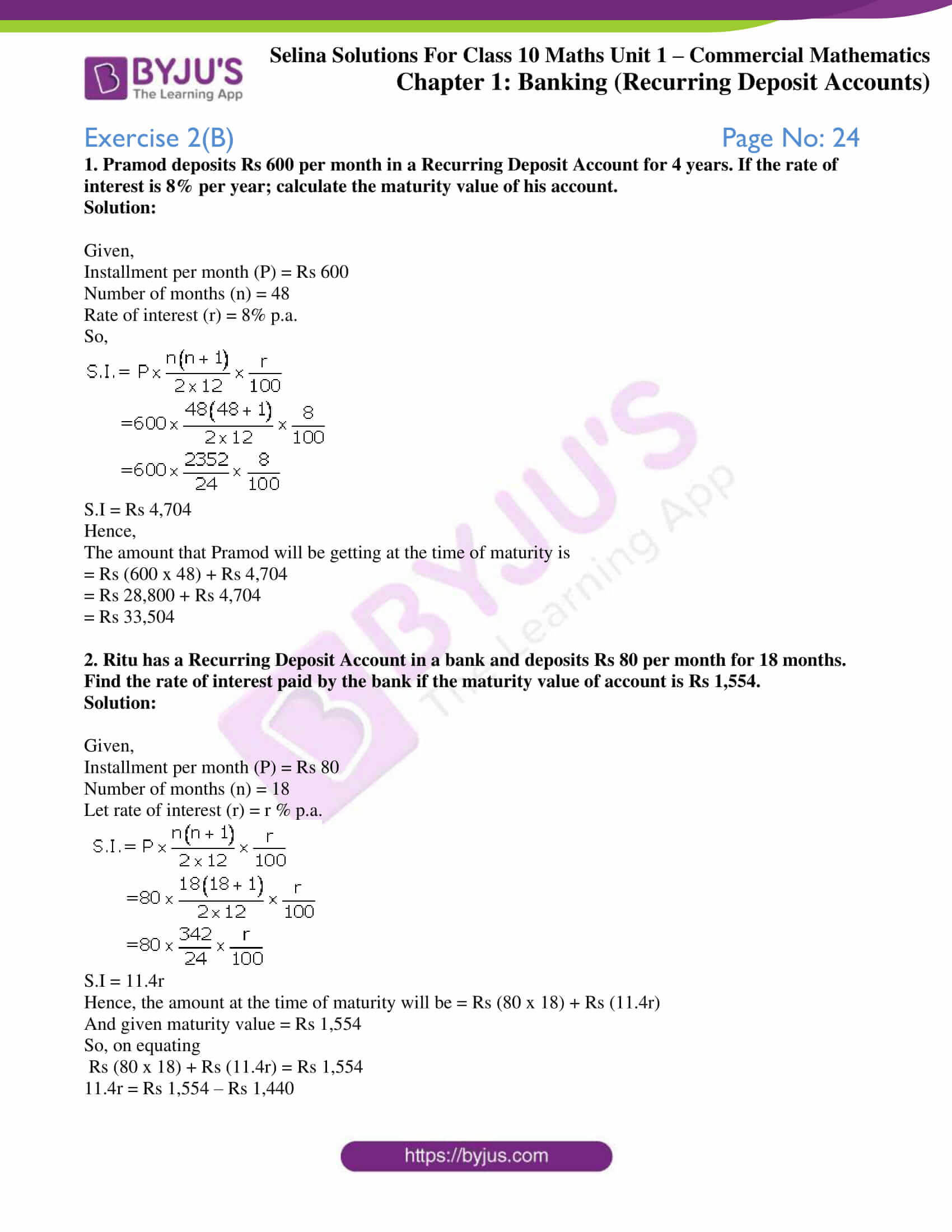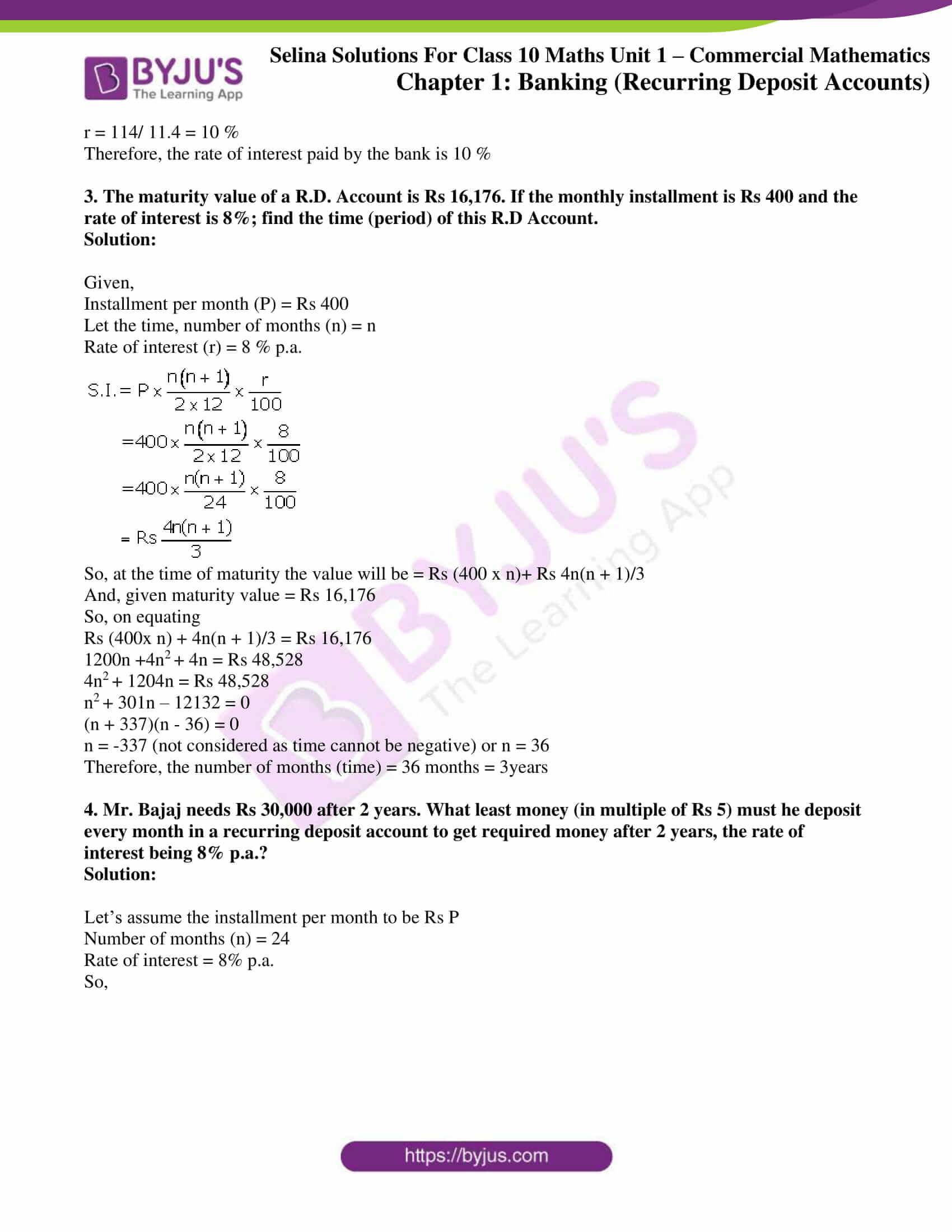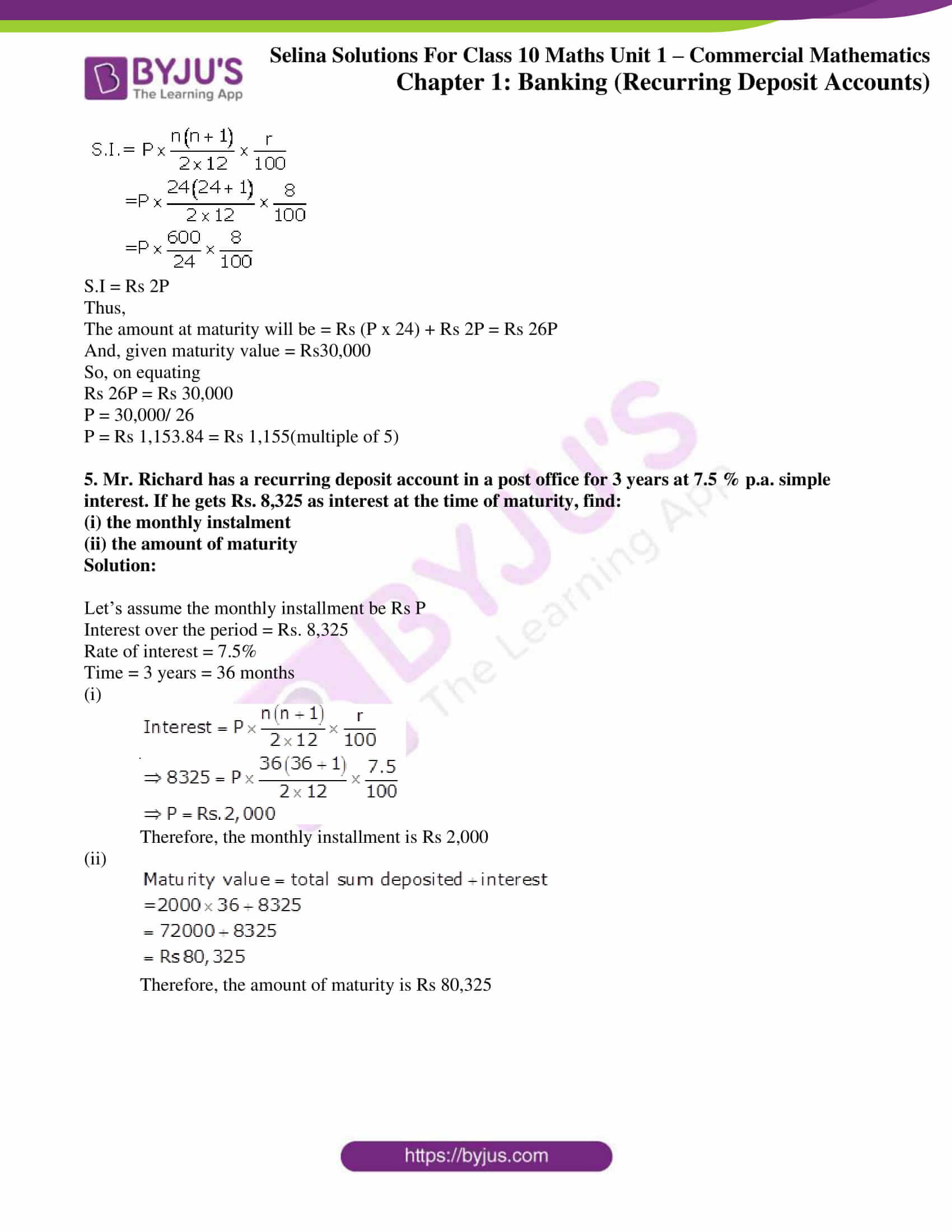### Exercise 2(B) Page No: 24

1. Pramod deposits Rs 600 per month in a Recurring Deposit Account for 4 years. If the rate of interest is 8% per year; calculate the maturity value of his account.

Solution:

Given,

Installment per month (P) = Rs 600

Number of months (n) = 48

Rate of interest (r) = 8% p.a.

So,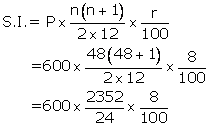S.I = Rs 4,704

Hence,

The amount that Pramod will be getting at the time of maturity is

= Rs (600 x 48) + Rs 4,704

= Rs 28,800 + Rs 4,704

= Rs 33,504

2. Ritu has a Recurring Deposit Account in a bank and deposits Rs 80 per month for 18 months. Find the rate of interest paid by the bank if the maturity value of account is Rs 1,554.

Solution:

Given,

Installment per month (P) = Rs 80

Number of months (n) = 18

Let rate of interest (r) = r % p.a.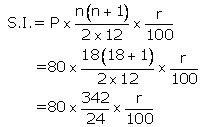S.I = 11.4r

Hence, the amount at the time of maturity will be = Rs (80 x 18) + Rs (11.4r)

And given maturity value = Rs 1,554

So, on equating

Rs (80 x 18) + Rs (11.4r) = Rs 1,554

11.4r = Rs 1,554 – Rs 1,440

r = 114/ 11.4 = 10 %

Therefore, the rate of interest paid by the bank is 10 %

3. The maturity value of a R.D. Account is Rs 16,176. If the monthly installment is Rs 400 and the rate of interest is 8%; find the time (period) of this R.D Account.

Solution:

Given,

Installment per month (P) = Rs 400

Let the time, number of months (n) = n

Rate of interest (r) = 8 % p.a.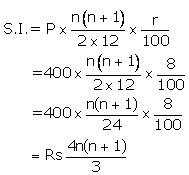So, at the time of maturity the value will be = Rs (400 x n)+ Rs 4n(n + 1)/3

And, given maturity value = Rs 16,176

So, on equating

Rs (400x n) + 4n(n + 1)/3 = Rs 16,176

1200n +4n2 + 4n = Rs 48,528

4n2 + 1204n = Rs 48,528

n2 + 301n – 12132 = 0

(n + 337)(n – 36) = 0

n = -337 (not considered as time cannot be negative) or n = 36

Therefore, the number of months (time) = 36 months = 3years

4. Mr. Bajaj needs Rs 30,000 after 2 years. What least money (in multiple of Rs 5) must he deposit every month in a recurring deposit account to get required money after 2 years, the rate of interest being 8% p.a.?

Solution:

Let’s assume the installment per month to be Rs P

Number of months (n) = 24

Rate of interest = 8% p.a.

So,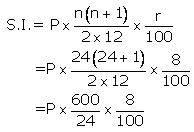S.I = Rs 2P

Thus,

The amount at maturity will be = Rs (P x 24) + Rs 2P = Rs 26P

And, given maturity value = Rs30,000

So, on equating

Rs 26P = Rs 30,000

P = 30,000/ 26

P = Rs 1,153.84 = Rs 1,155(multiple of 5)

5. Mr. Richard has a recurring deposit account in a post office for 3 years at 7.5 % p.a. simple interest. If he gets Rs. 8,325 as interest at the time of maturity, find:

(i) the monthly instalment

(ii) the amount of maturity

Solution:

Let’s assume the monthly installment be Rs P

Interest over the period = Rs. 8,325

Rate of interest = 7.5%

Time = 3 years = 36 months

(i)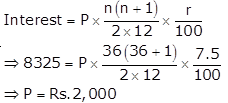Therefore, the monthly installment is Rs 2,000

(ii)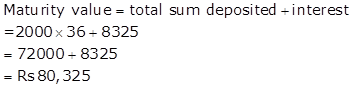Therefore, the amount of maturity is Rs 80,325

### Access another exercise of Selina Solutions Concise Maths Class 10 Chapter 2 Banking (Recurring Deposit Accounts)

Exercise 2(A) Solutions